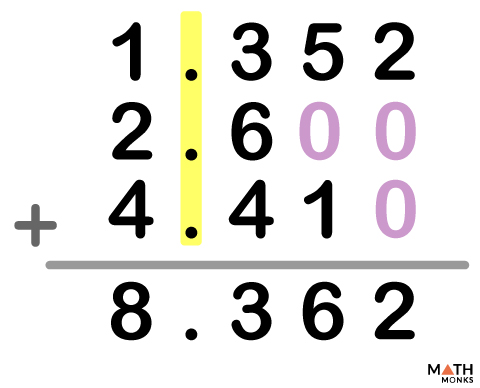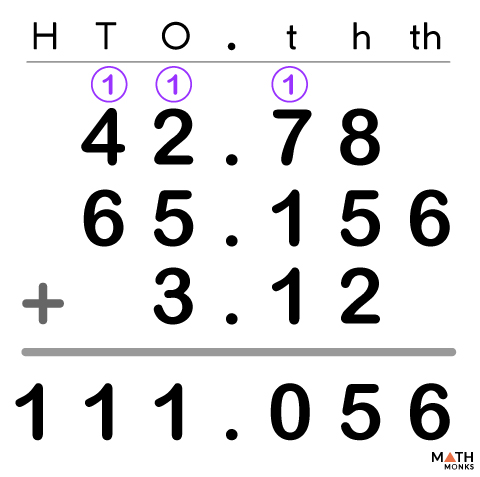### #ezw_tco-2 .ez-toc-widget-container ul.ez-toc-list li.active{ background-color: #ededed; } chapter outline

We not only add only whole numbers in our daily life, but also decimals when we carry out financial transactions, or when we cook or measure the size of our bathroom tiles.

An exmaple is shown in the diagram below where we involve three simple steps while adding like decimals (no. of digits after the decimal point is same).

1. Write down the decimal numbers one under the other, such that their decimals points are lined up.
2. Put extra zeros at the empty places to make the length of the numbers same.
4. Write down the result below. Drag the decimal point to the same position in the sum.

Let us add two unlike decimal numbers 49.365 and 7.2 involving the above four steps. The two numbers have different decimal places after the decimal point. The example is shown below:

Now let us add 0.3 and 0.52

0.3+0.52

Now we will make the lengths of the 2 numbers equal by adding ‘zero’ at the end.

So,

0.30+0.52

0.30+0.52

= 0.82

Add: 1.352 + 2.6 + 4.41.Solution:

Add: 7.3 + 6.343 + 8Solution:

As we know,
Here, the decimals numbers = 7.3 and 6.343,
And, the whole number = 8
We will put zeroes at their empty places in the decimal number and also in the whole number after the decimal point.